# Decrypt the encoded string with help of Matrix as per given encryption decryption technique

Given an encoded (or encrypted) string S of length N, an integer M. The task is to decrypt the encrypted string and print it. The encryption and decryption techniques are given as:

Encryption: The original string is placed in a Matrix of M rows and N/M columns, such that the first character of the Original text or string is placed on the top-left cell to the bottom-right manner. If last row is reached, then again go to the top row, and start from the next column.

For example: If string is “geeks”, M = 2, then the string will be encrypted in below manner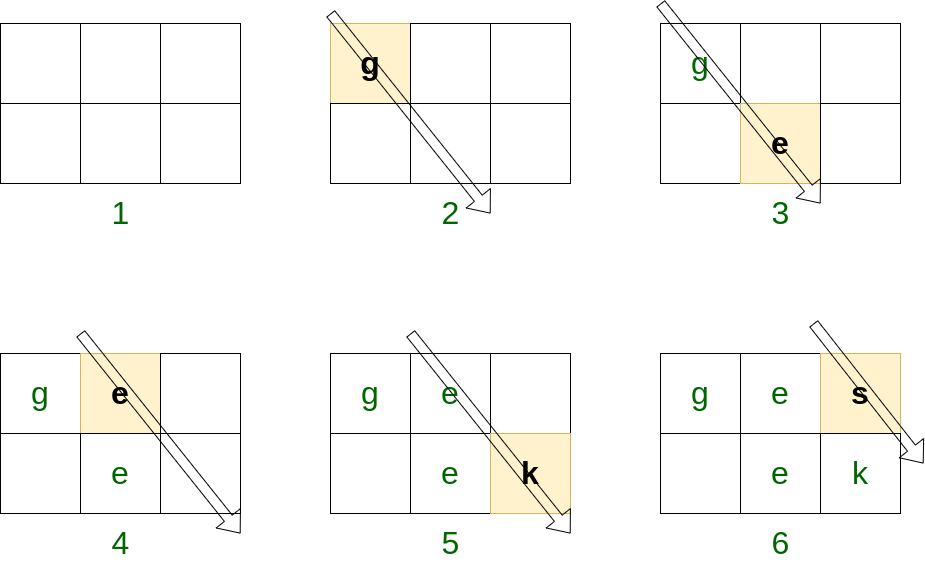Then traverse the matrix row wise and print the characters as they appear.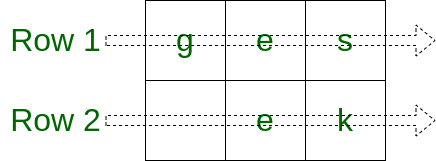Therefore the above string will be encrypted as = “ges ek”

Decryption: Traverse the matrix diagonally in the same manner as it was encrypted and find the actual string.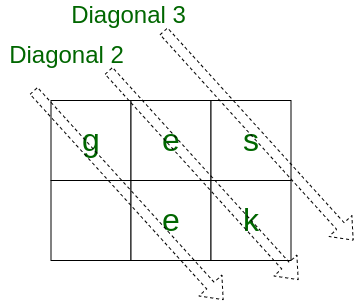Examples:

Input: “GSRE_ _ _E_ _K_ _ _EFGS_ _ _KOE” (Here ‘_’ means a space), 4
Output: “GEEKS FOR GEEKS”
Explanation:  See the image below for understanding the approach.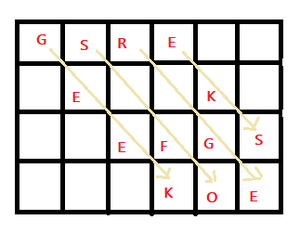Here column number is 6.
So, the traversing starts from (0, 0) position, then goes upto the end of the row, means from (0, 0) –> (1, 1)–>(2, 2)–>(3, 3)–>(4, 4). T
hen get to the first row, and start from the next, means from (0, 1)–>(1, 2)–>(2, 3)->(3, 4) and continues upto it reaches to the end of the given string.

Input: “GEEKSFORGEEKS”, 1
Output: “GEEKSFORGEEKS”
Explanation: There is only one row. so the decoded string will be as same as the given encoded string.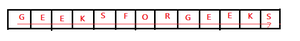Input: “abc de”, 2

Approach: First, find the number of columns. Then, start traversing. Follow the below steps to solve the problem:

• For each of the columns, go up to the length of the string starting from the i’th row, and after each traversal increase the iterating value to column+1.
• Because, here traversal is done diagonally, and here the next diagonal character will be after column number plus one.

For example, in the below picture, the X is a character, whose next diagonal character is XX, and the column number where X is present is 2, the next character row number is just one greater than the previous.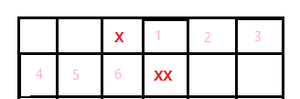• At the time of traversing add the characters into an empty string.  Then check, if there is any space at the end of the string or not, if it is, then just delete it.

At last, print that string, and this will be the decoded string/desired string.

Below is the implementation of the above approach:

## C++

 `// C++ program for the above approach` `#include ` `using` `namespace` `std;`   `// Function to find the desire string` `string decodeString(string encodedText, ``int` `rows)` `{`   `    ``// Stores the length of the string` `    ``int` `len = encodedText.size();`   `    ``// Stores the number of columns` `    ``int` `cols = len / rows;`   `    ``// Declaring an empty string` `    ``string res;` `    ``for` `(``int` `i = 0; i < cols; ++i)` `        ``for` `(``int` `j = i; j < len; j += cols + 1)` `            ``// Adding the characters` `            ``// into the empty string` `            ``res += encodedText[j];`   `    ``// If their any space at the end,` `    ``// delete it` `    ``while` `(res.back() == ``' '``) {` `        ``res.pop_back();` `    ``}` `    ``return` `res;` `}`   `// Driver Code` `int` `main()` `{`   `    ``string S = ``"GSRE   E  K   EFGS   KOE"``;` `    ``int` `row = 4;` `    ``cout << decodeString(S, row) << endl;` `    ``return` `0;` `}`

## Java

 `// Java program for the above approach` `class` `GFG {`   `  ``// Function to find the desire string` `  ``static` `String decodeString(String encodedText, ``int` `rows) {`   `    ``// Stores the length of the string` `    ``int` `len = encodedText.length();`   `    ``// Stores the number of columns` `    ``int` `cols = len / rows;`   `    ``// Declaring an empty string` `    ``String res = ``""``;` `    ``for` `(``int` `i = ``0``; i < cols; ++i) ` `    ``{` `      ``for` `(``int` `j = i; j < len; j += cols + ``1``)` `      ``{` `        ``// Adding the characters` `        ``// into the empty string` `        ``res += encodedText.charAt(j);` `      ``}` `    ``}`   `    ``// If their any space at the end,` `    ``// delete it` `    ``while` `(res.charAt(res.length() - ``1``) == ``' '``) {` `      ``res = res.substring(``0``, res.length() - ``2``);` `    ``}` `    ``return` `res;` `  ``}`   `  ``// Driver Code` `  ``public` `static` `void` `main(String args[]) {` `    ``String S = ``"GSRE   E  K   EFGS   KOE"``;` `    ``int` `row = ``4``;`   `    ``System.out.println(decodeString(S, row));`   `  ``}` `}`   `// This code is contributed by gfgking`

## Python3

 `# Python code for the above approach `   `# Function to find the desire string` `def` `decodeString(encodedText, rows):`   `    ``# Stores the length of the string` `    ``_len ``=` `len``(encodedText)`   `    ``# Stores the number of columns` `    ``cols ``=` `_len ``/``/` `rows`   `    ``# Declaring an empty string` `    ``res ``=` `"";` `    ``for` `i ``in` `range``(cols):` `        ``for` `j ``in` `range``(i, _len, cols ``+` `1``):` `        `  `            ``# Adding the characters` `            ``# into the empty string` `            ``res ``+``=` `encodedText[j];`   `    ``# If their any space at the end,` `    ``# delete it` `    ``while` `(res[``len``(res) ``-` `1``] ``=``=` `' '``):` `        ``res ``=` `res[``0``: ``len``(res) ``-` `1``];` `    ``return` `res;`     `# Driver Code` `S ``=` `"GSRE   E  K   EFGS   KOE"``;` `row ``=` `4``;` `print``(decodeString(S, row))`   `# This code is contributed by Saurabh Jaiswal`

## C#

 `// C# program for the above approach` `using` `System;` `class` `GFG` `{`   `// Function to find the desire string` `static` `string` `decodeString(``string` `encodedText, ``int` `rows)` `{`   `    ``// Stores the length of the string` `    ``int` `len = encodedText.Length;`   `    ``// Stores the number of columns` `    ``int` `cols = len / rows;`   `    ``// Declaring an empty string` `    ``string` `res = ``""``;` `    ``for` `(``int` `i = 0; i < cols; ++i) {` `        ``for` `(``int` `j = i; j < len; j += cols + 1) {` `            ``// Adding the characters` `            ``// into the empty string` `            ``res += encodedText[j];` `        ``}` `    ``}` ` `  `    ``// If their any space at the end,` `    ``// delete it` `    ``while` `(res[res.Length - 1] == ``' '``) {` `        ``res = res.Remove(res.Length - 1);` `    ``}` `    ``return` `res;` `}`   `// Driver Code` `public` `static` `void` `Main()` `{` `    ``string` `S = ``"GSRE   E  K   EFGS   KOE"``;` `    ``int` `row = 4;`   `    ``Console.Write(decodeString(S, row));`   `}` `}`   `// This code is contributed by Samim Hossain Mondal.`

## Javascript

 ``

Output

`GEEKS FOR GEEKS`

Time Complexity: O(M*(M/col)) which is near about O(length of the string)
Auxiliary Space: O(N)

Feeling lost in the world of random DSA topics, wasting time without progress? It's time for a change! Join our DSA course, where we'll guide you on an exciting journey to master DSA efficiently and on schedule.
Ready to dive in? Explore our Free Demo Content and join our DSA course, trusted by over 100,000 geeks!

Previous
Next